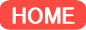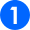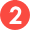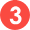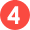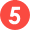The project is to publish Advances in Mathematical Economics once a year under the auspices of the Research Center for Mathematical Economics. It is designed to bring together those mathematicians who are seriously interested in obtaining new challenging stimuli from economic theories and those economists who are seeking effective mathematical tools for their research.

The scope of Advances in Mathematical Economics includes, but is not limited to, the following fields:
• Economics theories in various fields based on rigorous mathematical resoning.
• Mathematical methods (e.g., analysis, algebra, geometry, probability) motivated by economic theories.
• Mathematical results of potential relevance to economic theory.
• Historical study of mathematical economics.

Authors are asked to develop their original results as fully as possible and also to give a clear-cut expository overview of the problem under discussion. Consequently, we will also invite articles which might be considered too long for publication in journals.